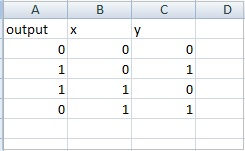# PyBrain - Importing Data For Datasets

In this chapter, we will learn how to get data to work with Pybrain datasets.

The most commonly used are datasets are −

• Using sklearn
• From CSV file

## Using sklearn

Using sklearn

Here is the link that has details of datasets from sklearn:https://scikit-learn.org/stable/datasets/index.html

Here are a few examples of how to use datasets from sklearn −

from sklearn import datasets
from pybrain.datasets import ClassificationDataSet
X, y = digits.data, digits.target
ds = ClassificationDataSet(64, 1, nb_classes=10)
for i in range(len(X)):


from sklearn import datasets
from pybrain.datasets import ClassificationDataSet
X, y = digits.data, digits.target
ds = ClassificationDataSet(4, 1, nb_classes=3)
for i in range(len(X)):


## From CSV file

We can also use data from csv file as follows −

Here is sample data for xor truth table: datasettest.csvHere is the working example to read the data from .csv file for dataset.

## Example

from pybrain.tools.shortcuts import buildNetwork
from pybrain.structure import TanhLayer
from pybrain.datasets import SupervisedDataSet
from pybrain.supervised.trainers import BackpropTrainer
import pandas as pd

data = df.values

train_output = data[:,0]
train_data = data[:,1:]

print(train_output)
print(train_data)

# Create a network with two inputs, three hidden, and one output
nn = buildNetwork(2, 3, 1, bias=True, hiddenclass=TanhLayer)

# Create a dataset that matches network input and output sizes:
_gate = SupervisedDataSet(2, 1)

# Create a dataset to be used for testing.
nortrain = SupervisedDataSet(2, 1)

# Add input and target values to dataset
# Values for NOR truth table
for i in range(0, len(train_output)) :

#Training the network with dataset norgate.
trainer = BackpropTrainer(nn, _gate)

# will run the loop 1000 times to train it.
for epoch in range(1000):
trainer.train()
trainer.testOnData(dataset=_gate, verbose = True)


Panda is used to read data from csv file as shown in the example.

## Output

C:\pybrain\pybrain\src>python testcsv.py
[0 1 1 0]
[
[0 0]
[0 1]
[1 0]
[1 1]
]
Testing on data:
('out: ', '[0.004 ]')
('correct:', '[0 ]')
error: 0.00000795
('out: ', '[0.997 ]')
('correct:', '[1 ]')
error: 0.00000380
('out: ', '[0.996 ]')
('correct:', '[1 ]')
error: 0.00000826
('out: ', '[0.004 ]')
('correct:', '[0 ]')
error: 0.00000829
('All errors:', [7.94733477723902e-06, 3.798267582566822e-06, 8.260969076585322e
-06, 8.286246525558165e-06])
('Average error:', 7.073204490487332e-06)
('Max error:', 8.286246525558165e-06, 'Median error:', 8.260969076585322e-06)